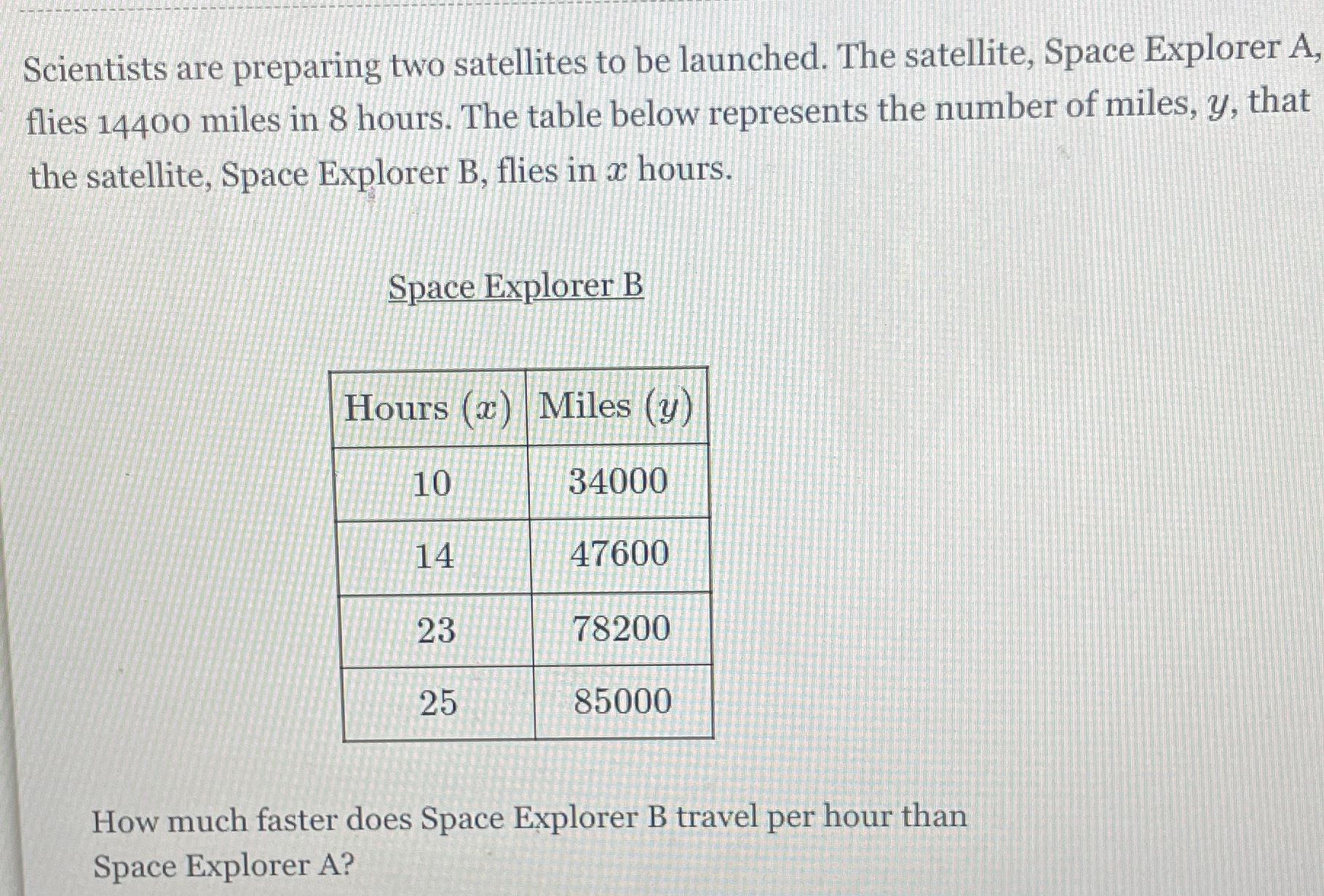### Still have math questions?

Algebra
QuestionScientists are preparing two satellites to be launched. The satellite, Space Explorer A, flies $$14400$$ miles in $$8$$ hours. The table below represents the number of miles, $$y$$ , that the satellite, Space Explorer B, flies in $$x$$ hours.

How much faster does Space Explorer B travel per hour than Space Explorer A?